# 2.2 Graphs of linear functions  (Page 5/15)

 Page 5 / 15

Find the x -intercept of $f\left(x\right)=\frac{1}{4}x-4.$

## Describing horizontal and vertical lines

There are two special cases of lines on a graph—horizontal and vertical lines. A horizontal line indicates a constant output, or y -value. In [link] , we see that the output has a value of 2 for every input value. The change in outputs between any two points, therefore, is 0. In the slope formula, the numerator is 0, so the slope is 0. If we use $m=0$ in the equation $f\left(x\right)=mx+b,$ the equation simplifies to $f\left(x\right)=b.$ In other words, the value of the function is a constant. This graph represents the function $f\left(x\right)=2.$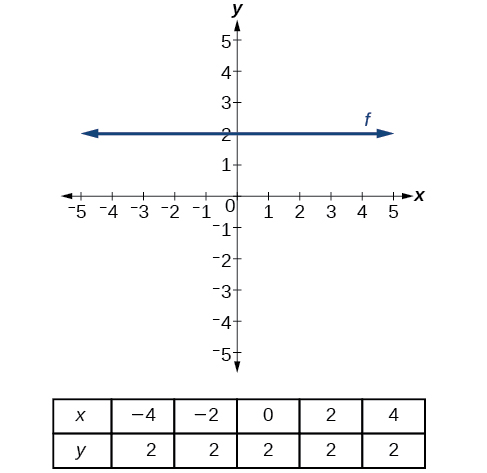A horizontal line representing the function f ( x ) = 2.

A vertical line indicates a constant input, or x -value. We can see that the input value for every point on the line is 2, but the output value varies. Because this input value is mapped to more than one output value, a vertical line does not represent a function. Notice that between any two points, the change in the input values is zero. In the slope formula, the denominator will be zero, so the slope of a vertical line is undefined.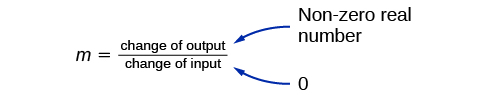Notice that a vertical line, such as the one in [link] , has an x -intercept, but no y- intercept unless it’s the line $x=0.$ This graph represents the line $x=2.$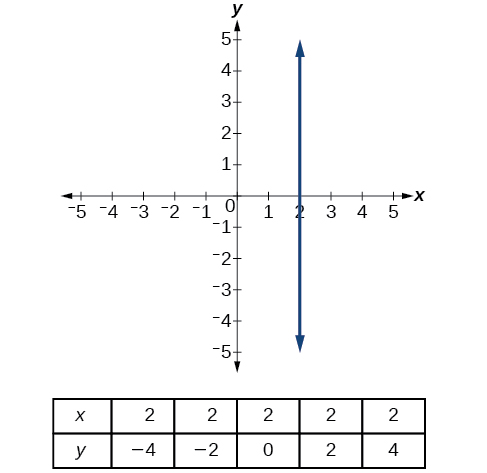The vertical line, x = 2 , which does not represent a function.

## Horizontal and vertical lines

Lines can be horizontal or vertical.

A horizontal line    is a line defined by an equation in the form $f\left(x\right)=b.$

A vertical line    is a line defined by an equation in the form $x=a.$

## Writing the equation of a horizontal line

Write the equation of the line graphed in [link] .

For any x -value, the y -value is $-4,$ so the equation is $y=-4.$

## Writing the equation of a vertical line

Write the equation of the line graphed in [link] .

The constant x -value is $7,$ so the equation is $x=7.$

## Determining whether lines are parallel or perpendicular

The two lines in [link] are parallel lines : they will never intersect. Notice that they have exactly the same steepness, which means their slopes are identical. The only difference between the two lines is the y -intercept. If we shifted one line vertically toward the y -intercept of the other, they would become the same line.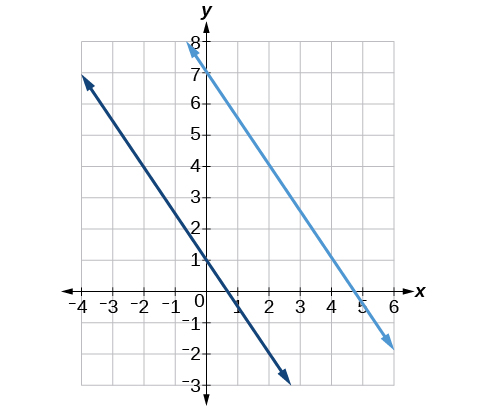Parallel lines.

We can determine from their equations whether two lines are parallel by comparing their slopes. If the slopes are the same and the y -intercepts are different, the lines are parallel. If the slopes are different, the lines are not parallel.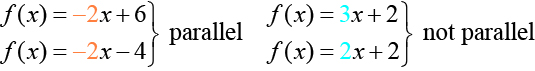Unlike parallel lines, perpendicular lines do intersect. Their intersection forms a right, or 90-degree, angle. The two lines in [link] are perpendicular.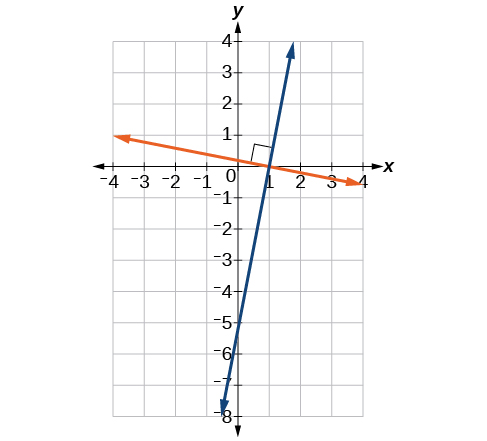Perpendicular lines.

Perpendicular lines do not have the same slope. The slopes of perpendicular lines are different from one another in a specific way. The slope of one line is the negative reciprocal of the slope of the other line. The product of a number and its reciprocal is $1.$ So, if are negative reciprocals of one another, they can be multiplied together to yield $–1.$

${m}_{1}{m}_{2}=-1$

To find the reciprocal of a number, divide 1 by the number. So the reciprocal of 8 is $\frac{1}{8},$ and the reciprocal of $\frac{1}{8}$ is 8. To find the negative reciprocal, first find the reciprocal and then change the sign.

#### Questions & Answers

How can you tell what type of parent function a graph is ?
Mary Reply
generally by how the graph looks and understanding what the base parent functions look like and perform on a graph
William
if you have a graphed line, you can have an idea by how the directions of the line turns, i.e. negative, positive, zero
William
y=x will obviously be a straight line with a zero slope
William
y=x^2 will have a parabolic line opening to positive infinity on both sides of the y axis vice versa with y=-x^2 you'll have both ends of the parabolic line pointing downward heading to negative infinity on both sides of the y axis
William
y=x will be a straight line, but it will have a slope of one. Remember, if y=1 then x=1, so for every unit you rise you move over positively one unit. To get a straight line with a slope of 0, set y=1 or any integer.
Aaron
yes, correction on my end, I meant slope of 1 instead of slope of 0
William
what is f(x)=
Karim Reply
I don't understand
Joe
Typically a function 'f' will take 'x' as input, and produce 'y' as output. As 'f(x)=y'. According to Google, "The range of a function is the complete set of all possible resulting values of the dependent variable (y, usually), after we have substituted the domain."
Thomas
Sorry, I don't know where the "Â"s came from. They shouldn't be there. Just ignore them. :-)
Thomas
GREAT ANSWER THOUGH!!!
Darius
Thanks.
Thomas
Â
Thomas
It is the Â that should not be there. It doesn't seem to show if encloses in quotation marks. "Â" or 'Â' ... Â
Thomas
Now it shows, go figure?
Thomas
what is this?
unknown Reply
i do not understand anything
unknown
lol...it gets better
Darius
I've been struggling so much through all of this. my final is in four weeks 😭
Tiffany
this book is an excellent resource! have you guys ever looked at the online tutoring? there's one that is called "That Tutor Guy" and he goes over a lot of the concepts
Darius
thank you I have heard of him. I should check him out.
Tiffany
is there any question in particular?
Joe
I have always struggled with math. I get lost really easy, if you have any advice for that, it would help tremendously.
Tiffany
Sure, are you in high school or college?
Darius
Hi, apologies for the delayed response. I'm in college.
Tiffany
how to solve polynomial using a calculator
Ef Reply
So a horizontal compression by factor of 1/2 is the same as a horizontal stretch by a factor of 2, right?
KARMEL Reply
The center is at (3,4) a focus is at (3,-1), and the lenght of the major axis is 26
Rima Reply
The center is at (3,4) a focus is at (3,-1) and the lenght of the major axis is 26 what will be the answer?
Rima
I done know
Joe
What kind of answer is that😑?
Rima
I had just woken up when i got this message
Joe
Can you please help me. Tomorrow is the deadline of my assignment then I don't know how to solve that
Rima
i have a question.
Abdul
how do you find the real and complex roots of a polynomial?
Abdul
@abdul with delta maybe which is b(square)-4ac=result then the 1st root -b-radical delta over 2a and the 2nd root -b+radical delta over 2a. I am not sure if this was your question but check it up
Nare
This is the actual question: Find all roots(real and complex) of the polynomial f(x)=6x^3 + x^2 - 4x + 1
Abdul
@Nare please let me know if you can solve it.
Abdul
I have a question
juweeriya
hello guys I'm new here? will you happy with me
mustapha
The average annual population increase of a pack of wolves is 25.
Brittany Reply
how do you find the period of a sine graph
Imani Reply
Period =2π if there is a coefficient (b), just divide the coefficient by 2π to get the new period
Am
if not then how would I find it from a graph
Imani
by looking at the graph, find the distance between two consecutive maximum points (the highest points of the wave). so if the top of one wave is at point A (1,2) and the next top of the wave is at point B (6,2), then the period is 5, the difference of the x-coordinates.
Am
you could also do it with two consecutive minimum points or x-intercepts
Am
I will try that thank u
Imani
Case of Equilateral Hyperbola
Jhon Reply
ok
Zander
ok
Shella
f(x)=4x+2, find f(3)
Benetta
f(3)=4(3)+2 f(3)=14
lamoussa
14
Vedant
pre calc teacher: "Plug in Plug in...smell's good" f(x)=14
Devante
8x=40
Chris
Explain why log a x is not defined for a < 0
Baptiste Reply
the sum of any two linear polynomial is what
Esther Reply
divide simplify each answer 3/2÷5/4
Momo Reply
divide simplify each answer 25/3÷5/12
Momo
how can are find the domain and range of a relations
austin Reply
the range is twice of the natural number which is the domain
Morolake
A cell phone company offers two plans for minutes. Plan A: $15 per month and$2 for every 300 texts. Plan B: $25 per month and$0.50 for every 100 texts. How many texts would you need to send per month for plan B to save you money?
Diddy Reply
6000
Robert
more than 6000
Robert
For Plan A to reach $27/month to surpass Plan B's$26.50 monthly payment, you'll need 3,000 texts which will cost an additional \$10.00. So, for the amount of texts you need to send would need to range between 1-100 texts for the 100th increment, times that by 3 for the additional amount of texts...
Gilbert
...for one text payment for 300 for Plan A. So, that means Plan A; in my opinion is for people with text messaging abilities that their fingers burn the monitor for the cell phone. While Plan B would be for loners that doesn't need their fingers to due the talking; but those texts mean more then...
Gilbert
can I see the picture
Zairen Reply

### Read also:

#### Get the best Precalculus course in your pocket!

Source:  OpenStax, Precalculus. OpenStax CNX. Jan 19, 2016 Download for free at https://legacy.cnx.org/content/col11667/1.6
Google Play and the Google Play logo are trademarks of Google Inc.

Notification Switch

Would you like to follow the 'Precalculus' conversation and receive update notifications?ByByBy